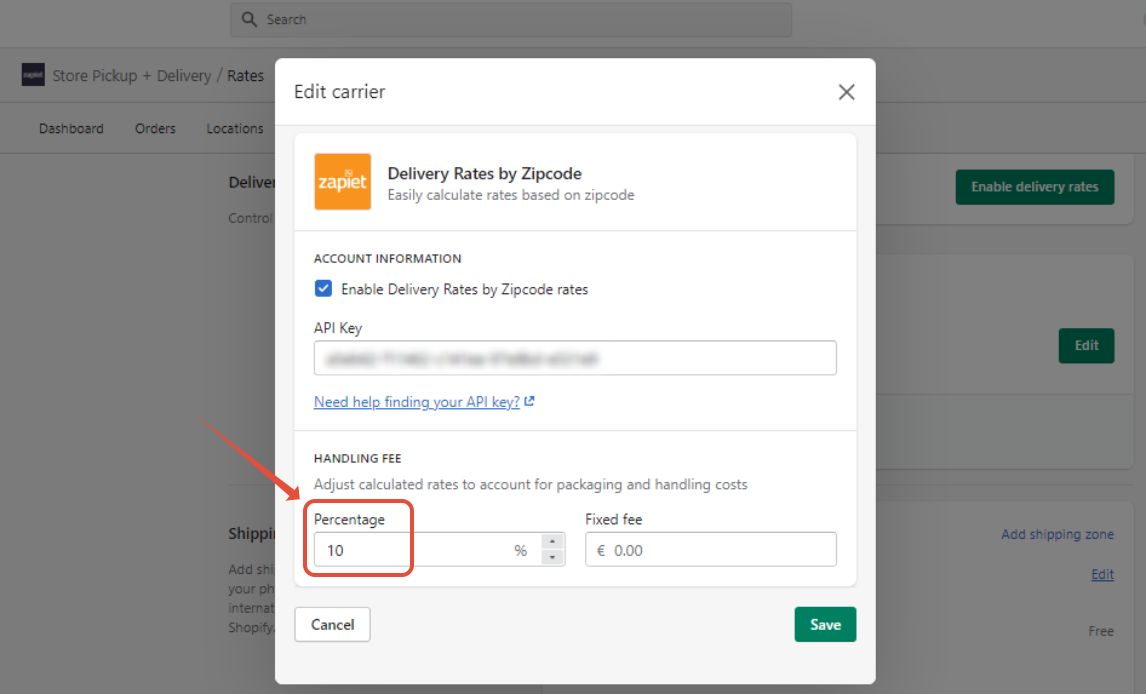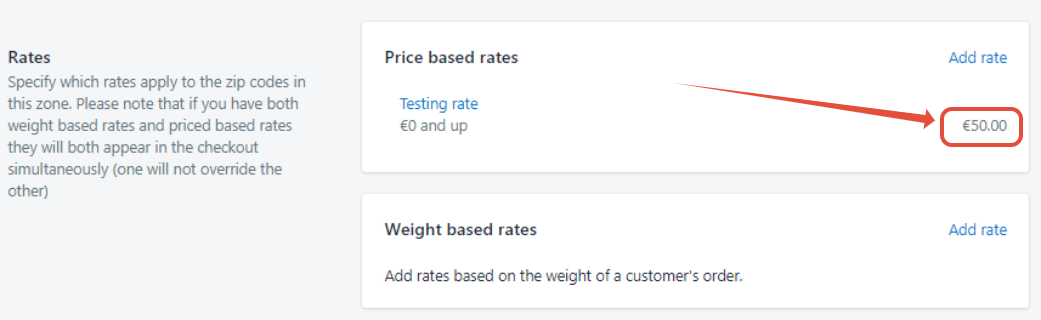# Calculating handling fees

Within Store Pickup + Delivery, you can integrate with carriers that calculate more complex rates. We provide the ability to add a percentage of the rate or a fixed fee to the rates provided by these apps.

### Percentage handling fee

This will take the rate provided by the carrier, and add a percentage of this rate to the shipping fee.

The final rate will be calculated by the following formula:

Final rate = original rate + the percentage of the rate

#### Example calculation

The percentage fee has been set as 10% of the original rate.In this example, the original rate is  50.Following the above formula, the rate the customer will pay is the original rate + the handling fee.

In this example, it is € 50 + (10% of € 50) = € 55.### Fixed handling fee

This will take the rate provided by the carrier, and add a fixed fee to the shipping fee.
The final rate will be calculated by the following formula:

Final rate =  original rate + the fixed fee

#### Example calculation

The fixed fee has been set to 10.The original rate has been set to 50.Following the above formula, the rate the customer will pay is the original fee + the fixed fee.

In this example, it is 50 + 10 = 60.### Percentage and fixed handling fees

If you add both a percentage and a fixed fee, the rate will be calculated by first finding the percentage of the original rate. That percentage will then be added to the original rate, along with the fixed fee.

The final rate will be calculated by the following formula:

Final rate = original rate + the percentage fee of the original rate + the fixed fee

#### Example calculation

The percentage fee has been set to 10% of the original fee, and the fixed fee is set as  3.The original rate is set as € 50.Following the above formula, the final rate will be the original fee + the percentage fee + the fixed fee.

In this example, this will be € 50 + (10% of € 50) + € 3 = € 58.### Percentage handling fees within Shopify

You can add a handling fee to Store Pickup + Delivery rates in Shopify, and it will be applied to all rates coming from Store Pickup + Delivery.

If you add a percentage handling fee in Shopify and in Store Pickup + Delivery, the handling fee will be added in Store Pickup + Delivery first, and then the Shopify handling fee is calculated from the Store Pickup + Delivery rate.

The Store Pickup + Delivery (SPD) rate will be calculated by the following formula:

SPD rate = carrier rate + percentage of the carrier rate + fixed fee

The final rate will be calculated by the following formula:

Final rate = SPD rate + percentage of the SPD rate

#### Example calculation

The SPD handling fee has been set to a 10% percentage fee.The original rate has been set to 50.Following the above formula, the SPD rate = 50 + (10% of € 50) = € 55.

The handling fee in Shopify is set to 100%.Following the above formula, the final rate = € 55 + (100% of € 55) = € 110.### Limitations

• The handling fees cannot be set as negative values. This means they can't be used to discount the rate.
• You cannot set percentage handling fees as a decimal—it will only accept whole numbers.

Did you find it helpful? Yes No

Send feedback